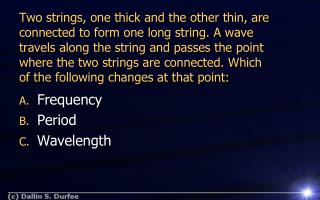DownloadDownload PresentationFrequency Period Wavelength

# Frequency Period Wavelength

Télécharger la présentation## Frequency Period Wavelength

- - - - - - - - - - - - - - - - - - - - - - - - - - - E N D - - - - - - - - - - - - - - - - - - - - - - - - - - -
##### Presentation Transcript

1. Two strings, one thick and the other thin, areconnected to form one long string. A wavetravels along the string and passes the pointwhere the two strings are connected. Whichof the following changes at that point: • Frequency • Period • Wavelength

2. Sound waves • Longitudinal • Speed of sound

3. Sound waves • Velocity of sound in air at 20o is 343 m/s • Helium is lighter than air • Velocity of sound in helium at 20o is 972 m/s

4. Sound tend to travel faster in liquids and solids (the molecules are closer together). • The velocity of sound in air vin air = 343 m/s • The velocity of sound in liquid vin water = 1490 m/s • The velocity of sound in a solid vin Aluminum = 5100m/s Cool experiment – make a milkshake!

5. Why do we not hear collisions and explosions from outer space? • In a vacuum there are not any molecules • Without molecules we cannot have a pressure wave • Sound cannot travel through a vacuum. Sound in a vacuumDemo

6. JOHN 14:27 Peace I leave with you, my peace I give to you: not as the world gives, give I to you. Let not your heart be troubled, neither let it be afraid.

7. Intensity of sound Intensity depends on __________ and the _____________ from the source. Power Distance

8. Spherical Waves / Plane Waves

9. Decibel Scale Zero decibels refers to the threshold of hearing

10. log (10x) = x log 10 = x • 10logx=x

11. log(ab)=log a + log b • log(a/b)=log a - log b

12. Decibel Scale Zero decibels refers to the threshold of hearing

13. At a rock concert a meter placed at 2 m in front of a loudspeaker measures 130 dB. What is the power output of the speaker?

14. At a rock concert a meter placed at 2 m in front of a loudspeaker measures 130 dB. How far away do I need to stand to be at a safer level of 90 dB?

15. If you increase the intensity by a factor of 1000, add ____ to the decibel level. 3 dB 10 dB 20 dB 30 dB 60 dB

16. If you increase the intensity by a factor of 2, add ____ to the decibel level. 3 dB 10 dB 20 dB 30 dB 60 dB

17. Can you detect a 3dB difference (50% loss) http://www.phys.unsw.edu.au/jw/hearing.html

18. Other Decibel Scales • Weighting • dBm • dBV • dB SPL • dBFV

19. Consider music from a radio. If I turn up the volume so that the sound level is 20 dB louder, by what factor has the intensity increased? (a) 10 (b) 20 (c) 100 Q15-2

20. When I sit one meter from a radio, the sound level is 80 dB. How far from the radio should I sit to reduce the sound level to 60 dB? (a) 3 m (b) 10 m (c) 30 m (d) 100 m (e) 300 m Q15-3